# Goodness-of-Fit Algorithms

## Goodness-of-Fit Algorithms

### Introduction

These web pages provide some information and results on goodness-of-fit tests for exponentiality, as described in Haywood and Khmaladze (2005). Algorithms using the R statistical software are also described. We wish to test the hypothesis that the sample {Xi}ni=1 is from an exponential distribution or, more formally, that the i.i.d. random variables {Xi}ni=1 all have exponential distribution function F(x)=1-e-λx for some unknown (or unspecified) value of λ>0.

### Distribution-free goodness-of-fit testing of exponentiality

We suggest as test statistics functionals from the transformed empirical process

wn(x) = √n[Pn(x) - Kn(x, Pn)]

where Pn(x) is the empirical distribution function of the observations and Kn(x, Pn) is its compensator. In the case of exponential distributions the compensator has the very simple form: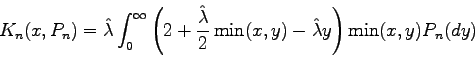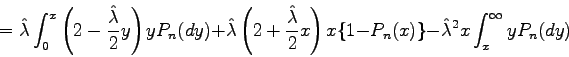or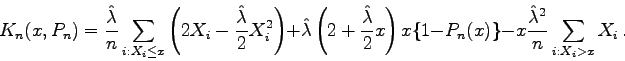### Calculation of Kolmogorov-Smirnov statistics for testing exponentiality via Khmaladze transformation

Calculation of the Kolmogorov-Smirnov statistics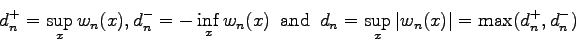becomes easy and yet exact, since within each interval (X(j), X(j+1)) formed by adjacent order statistics the compensator Kn(x, Pn) is a quadratic function in x.

A background note explains the theory behind these calculations. In the following calculations and code, the parameter lambda is estimated by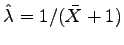. The code in R (see The R Project for Statistical Computing) for calculation of all three statistics takes the form of a series of functions shown below. We also show an example of Kn(x,Pn) for a small sample size (n=5).

### The R code

Each of the following R functions is vectorised in x.
```nPn <- function(x, X, n) {
# returns n*Pn(x) given data X
lx <- length(x)
numi <- numeric(lx)
for (i in 1:lx)
numi[i] <- sum(X <= x[i])
return(numi)
}

K <- function(x, X, n) {
# returns K(x, Pn(x)) given data X
lhat <- 1/(mean(X) + 1)
cX <- c(0, cumsum(X))
cX2 <- c(0, cumsum(X**2))
crX <- cX[n+1] - cX
if ((lx <- length(x)) > 0) {
whichn <- nPn(x, X, n) + 1
}
return(lhat/n*(2*cX[whichn] - lhat/2*cX2[whichn]) +
lhat*(2 + lhat/2*x)*x*(1 - nPn(x, X, n)/n) -
lhat**2*x/n*crX[whichn])
}

x0 <- function(X, n) {
# returns the value of x0 for each interval of X,
# or the interval boundary value closest to it
cX <- cumsum(X)
crX <- cX[n] - cX[-n]
invlhat <- mean(X) + 1
maxloc <- crX/((n - 1):1) - 2*invlhat
return(pmin(X[-1], pmax(X[-n], maxloc)))
}

findSup <- function(X) {
n <- length(X)
ivec <- 1:n
ishort <- 1:(n - 1)
dplus <- max(ivec/n - K(X, X, n), ishort/n - K(x0(X, n), X, n))
dminus <- -min((ivec - 1)/n -  K(X, X, n))
return(list(dplus=dplus, dminus=dminus, d=max(dplus, dminus)))
}
```

The Kolmogorov-Smirnov statistic is found by typing the R command:

```findSup(sort(mydata))
```
where `mydata` is the dataset of interest.

In the small sample graph [PDF, 37KB], the compensator Kn(x, Pn) in black has one interval where it attains its minimum within the two endpoints of the interval (rather than at one of the endpoints). This is actually a rare occurrence, occurring perhaps only once in 1000 intervals.